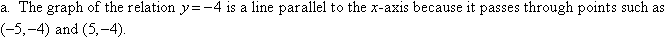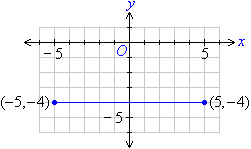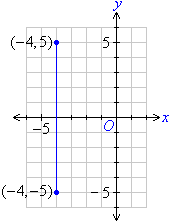Year 8 Interactive Maths - Second Edition

## Horizontal and Vertical Lines

The graph of a relation of the form y = 5 is a line parallel to the x-axis because the y value never changes.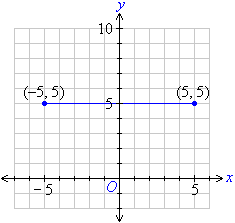###### Note:

A line parallel to the x-axis is called a horizontal line.

The graph of a relation of the form x = 5 is a line parallel to the y-axis because the x value never changes.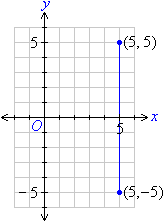###### Note:

A line parallel to the y-axis is called a vertical line.

#### Example 8

Plot the graph of each of the following relations: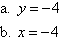##### Solution: